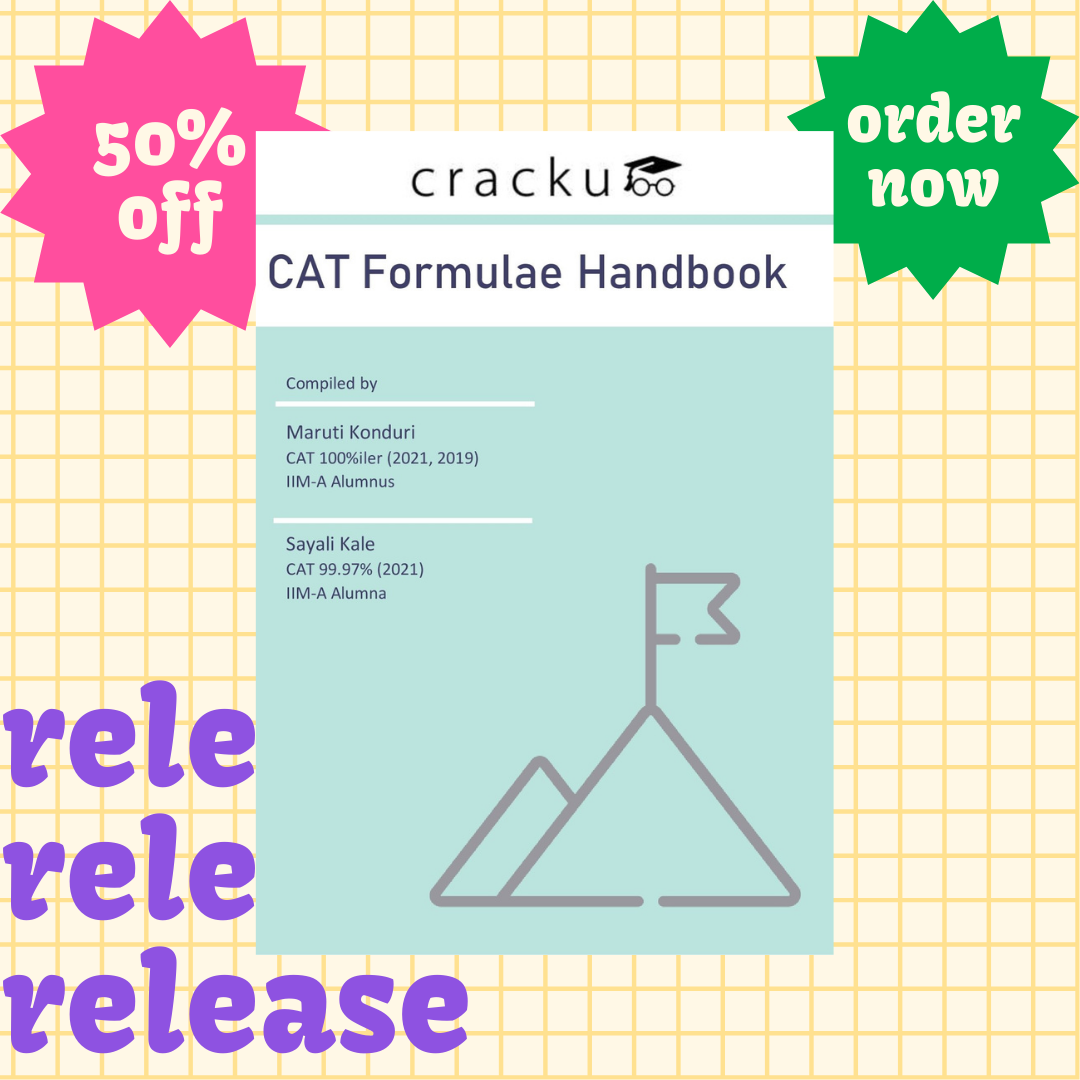# Number Systems Formulas For CAT PDF

0
96698

Number Systems is one of the important topics in the quantitative section. It is a very vast topic and a significant number of questions appear in CAT every year from this section. Learning simple tricks like divisibility rules, HCF and LCM, prime number and remainder theorems can help improve the score drastically. This pdf presents best shortcuts which make this topic easy and helps you perform better.

Checkout CAT Number systems questions in previous papers

Looking for hardcopy handbook?

Order below. Delivery charges are on us 🙂Outline of various topics covered are

• L.C.M and H.C.F formulas
• Prime and Composite numbers
• Properties of Prime Numbers
• Theorems on Prime numbers (Fermat's Theorem, Wilson's TheoremRemainder Theorem for CAT, Euler's Theorem)
• Highest power of a number in for a factorial number
• To find the number of zeroes in n!
• Number of factors
• Sum of the factors
• number of even and odd factors
• Number of positive integral solutions and some other related formulas
• Divisibility of numbers
• Divisibility properties
• Cyclicity and many more formulas on number systems

You can practice form Number systems questions for CAT PDF.

Take a free CAT mock test. Practice number systems questions in CAT Study Room.